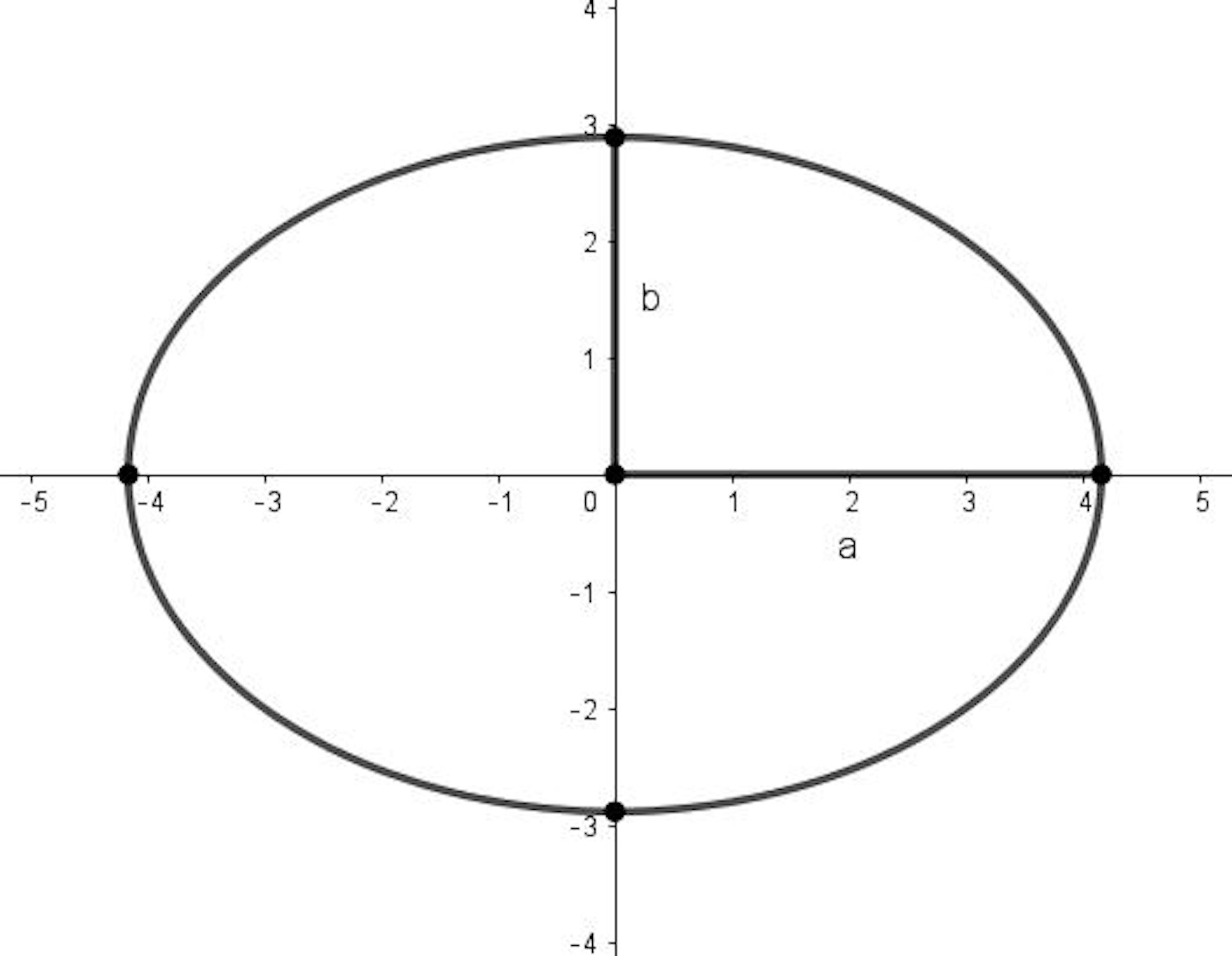The Colosseum: a well-known ellipse. Diliff/wikipedia, CC BY-SA

A lot of people claim they don’t have a “maths brain”. But the truth is that mathematicians think in all sorts of ways. There’s no one right way to think about maths – and that can create communication challenges, if someone thinking with a visual image is trying to share ideas with someone who is trying to use an equation. But it’s also immensely powerful. And if you’re finding it hard to understand a piece of maths, then maybe it’s worth looking from another perspective.

Take ellipses, for example. If you look around you, you’ll probably be able to see an ellipse, such as the rim of a coffee cup. There are a lot of ways to think about ellipses. Here’s a quick guided tour to see how different mathematicians might have different perspectives.

## Think practically

In the same way as you can use a stick and a piece of string to draw a circle where all the points are at fixed distance from the centre, you can use sticks and a loop of string to mark out an ellipse. To do this, plant two sticks in the ground and place the loop around the two sticks and use a third stick to pull the string tight. Now drag this stick around to mark out the ellipse. As long as the string is tight, the stick will move in an ellipse – you can feel the tension in the string guiding its motion.

The practical act of drawing an ellipse gives a compelling way to get a feeling for it.

## Think visually

Ellipses belong to a family of curves called “conic sections”. These are the curves obtained by slicing a hollow cone at various angles. There are several types of conic section: the ellipse and circle are two (or possibly just one, since a circle is a special type of ellipse), but we can also obtain a parabola and a hyperbola.

With this visual way of thinking about an ellipse, we can think of it as just one of a family of related curves. We can then seek to understand general conic sections and later specialise to the ellipse. This involves an up-front investment of time, but pays off later when we have a general theory that applies to several types of curve rather than just one.

## Think in words

Not everyone prefers visual thinking, even for geometrical ideas. Some people may prefer to think about mathematical concepts in words, or it may be more convenient to communicate a particular idea in words. Our mathematics undergraduates are often surprised to reach university and discover how many words we expect to see in their solutions. Mathematical arguments are often best described by sentences that tell a coherent story, with equations and diagrams included at appropriate points.

We’ve described ellipses in various ways in this article, and these bring out different features of an ellipse, so we might wonder how can we be sure that these all describe the same type of curve. We might also want to make some of these descriptions more precise.

A defining property of an ellipse is that, for each point on it, the sum of the distances from the point to the two focal points (the two sticks in the drawing) is constant. We could even say this more precisely: an ellipse is a curve in a plane surrounding two focal points such that the sum of the distances to the two focal points is constant for every point on the curve. This description may be enough for some purposes, but people might try to interpret what it means visually, or to test it out to see how it relates to a particular ellipse or to turn the description into an equation.

## Think algebraically

Some people like to turn geometry problems into algebra. This can lead to different sorts of insights. Someone who likes to think in symbols might capture the idea of an ellipse by saying that PS+PS’=C (where C is a constant).

It doesn’t end there. A circle drawn on a coordinate system with zero at the centre and radius 1 has the equation x2 + y2 = 1. Ellipses behave differently, because for most ellipses the distance from the centre to the curve is not constant. It varies between two values (a and b in the diagram).Ellipse with axes a and b.

The lengths a and b define how large and how “elliptical” the ellipse is, and give us an equation of the ellipse: (x/a)2 + (y/b)2 = 1. This is intimately connected with the equation of a circle – we can get an ellipse by stretching a circle. That’s how we got the equation above.

Equations for curves can be really helpful for exploring relationships with other geometrical objects with nice equations. For example, to find the points where a line intersects an ellipse, we can write down the equations of both, and solve to find the coordinates.

## Think your own way

Finding your own way to think about mathematical concepts is absolutely key – whether you do that by counting fingers, making drawings or saying things out loud. Progress is often made in mathematical research because someone has found a new way to explore an idea.

All mathematicians have their own favourite ways to think about mathematical problems. Some people instinctively prefer one sort of approach over another – which may be down to habit or having had previous success with one approach or struggled with another. Perhaps the most successful mathematicians are those who are able to flip fluently between approaches, as some strategies work better than others for any given problem.

### Want to write?

Write an article and join a growing community of more than 89,800 academics and researchers from 2,958 institutions.